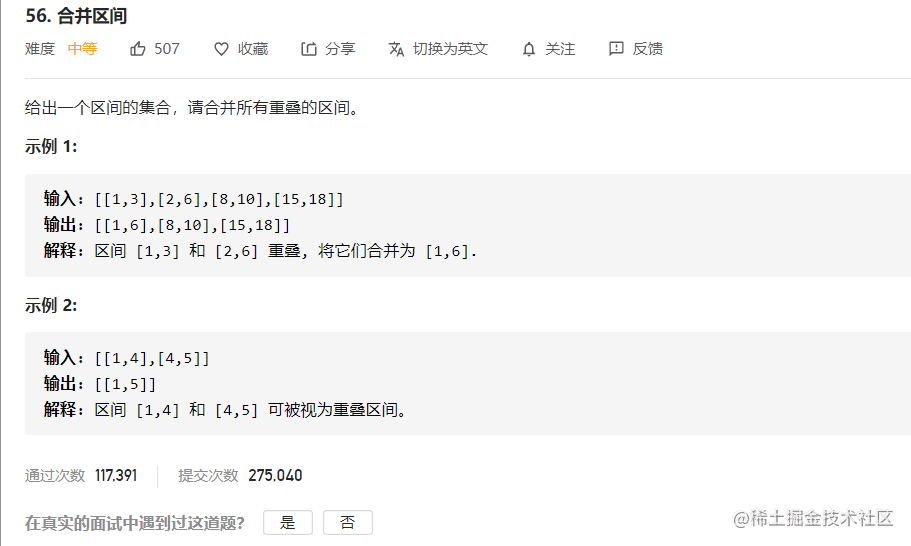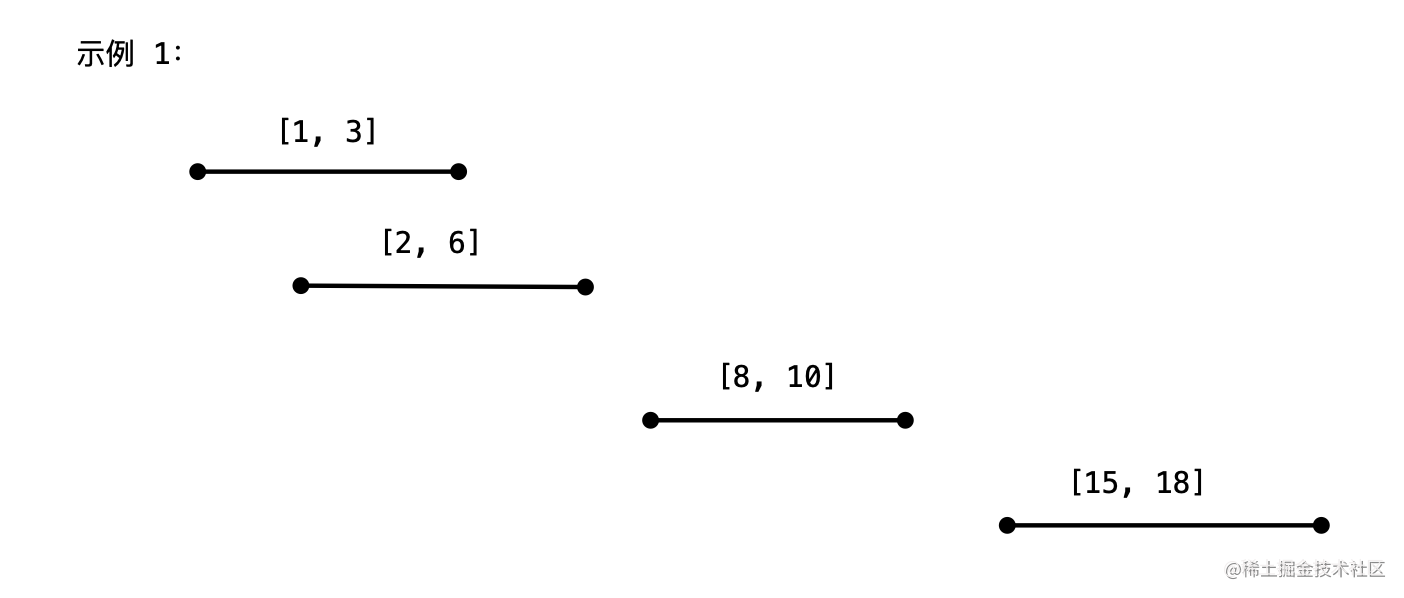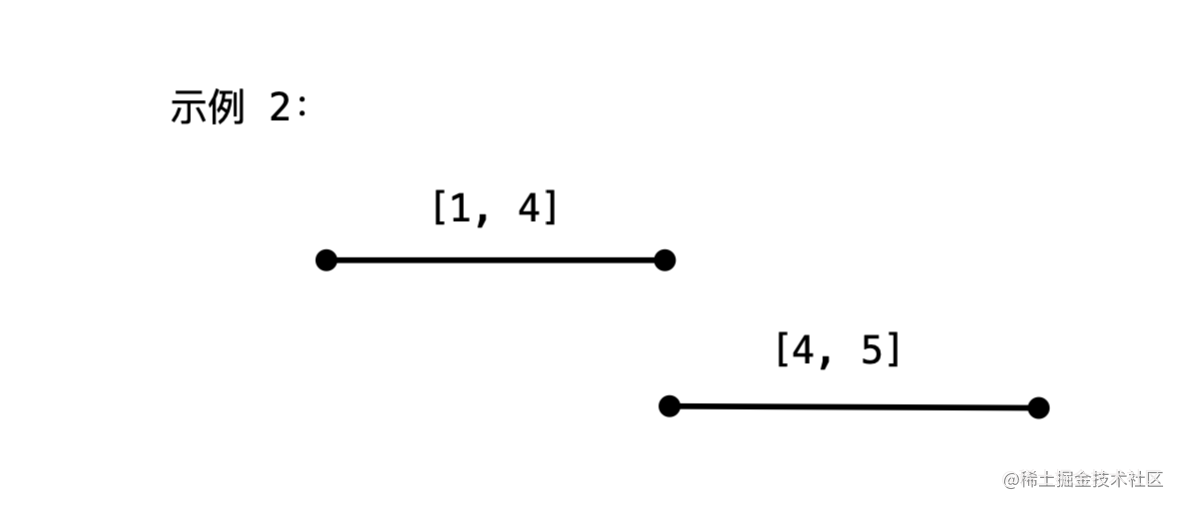# 题目# 分析

### 方法一：贪心算法

• 首先画图理解题意；• 和物理现象相关的：第 42 题：接雨水问题、第 11 题：盛最多水的容器、第 218 题：天际线问题；
• 本身问题描述就和图形相关的问题：第 84 题：柱状图中最大的矩形；
• 链表问题：穿针引线如果不画图容易把自己绕晕；
• 回溯算法问题：根据示例画图发现每一步的选择和剪枝的条件；
• 动态规划问题：画示意图发现最优子结构。

• 如果当前遍历到的区间的左端点 > 结果集中最后一个区间的右端点，说明它们没有交集，此时把区间添加到结果集；
• 如果当前遍历到的区间的左端点 <= 结果集中最后一个区间的右端点，说明它们有交集，此时产生合并操作，即：对结果集中最后一个区间的右端点更新（取两个区间的最大值）。

• Java
import java.util.ArrayList;
import java.util.Arrays;
import java.util.Collections;
import java.util.Comparator;
import java.util.List;
import java.util.Stack;

public class Solution {

public int[][] merge(int[][] intervals) {
int len = intervals.length;
if (len < 2) {
return intervals;
}

// 按照起点排序
Arrays.sort(intervals, Comparator.comparingInt(o -> o));

// 也可以使用 Stack，因为我们只关心结果集的最后一个区间
List<int[]> res = new ArrayList<>();

for (int i = 1; i < len; i++) {
int[] curInterval = intervals[i];

// 每次新遍历到的列表与当前结果集中的最后一个区间的末尾端点进行比较
int[] peek = res.get(res.size() - 1);

if (curInterval > peek) {
} else {
// 注意，这里应该取最大
peek = Math.max(curInterval, peek);
}
}
return res.toArray(new int[res.size()][]);
}

public static void main(String[] args) {
Solution solution = new Solution();
int[][] intervals = {{1, 3}, {2, 6}, {8, 10}, {15, 18}};
int[][] res = solution.merge(intervals);
for (int i = 0; i < res.length; i++) {
System.out.println(Arrays.toString(res[i]));
}
}
}

• 时间复杂度：O*(Nlog*N)，这里 N是区间的长度；
• 空间复杂度：O*(*N)，保存结果集需要的空间，这里计算的是最坏情况，也就是所有的区间都没有交点的时候。

• 前提：区间按照左端点排序；
• 贪心策略：在右端点的选择中，如果产生交集，总是将右端点的数值更新成为最大的，这样就可以合并更多的区间，这种做法是符合题意的。

• 直觉，根据直觉描述出来的算法，具备「只考虑当前，不考虑全局」的特点，那可能就是「贪心算法」；
• 如果不能举出反例，那多半这个问题就具有「贪心算法性质」，可以使用贪心算法去做。

• 数学归纳法；
• 反证法。

• 当前决策对后面的决策不产生影响；
• 当前决策只需要记录一个结果，而这个决策是怎么来的不重要。

### 贪心算法的应用

• 对数据压缩编码的霍夫曼编码（Huffman Coding）
• 求最小生成树的 Prim 算法和 Kruskal 算法
• 求单源最短路径的Dijkstra算法